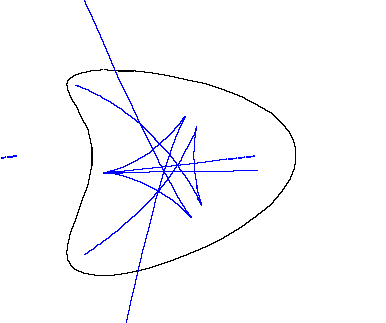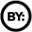A Program for calculating singular algebraic curves and surfaces
R Morris 1990-2003

# SingSurfPro: 3D curves and surface visualizerA Symmetry Set

SingSurfPro and SingSurf2D are programs to visulise many of the curves and surfaces which can occur in Singularity Theory and related subjects. It specalises in drawing more complex types of surface which contain singular points like cusps.

SingSurf can draw:

Algebraic Surfaces (asurf)
Surfaces defined as the zero set of a polynomial in x,y,z e.g. a sphere x^2+y^2+z^2=1;. examples
Algebraic Curves (acurve)
Curves defined by a polynomial in x,y e.g. a circle x^2+y^2=1;. examples
Parameterised Surfaces (psurf)
Surfaces defined by a parameterised equations in two variables. e.g. a sphere [cos(x) cos(y), cos(x) sin(y),sin(x)];. examples
Parameterised Curves (pcurve)
Curves defined by a parameterised vector equations in one variables. e.g. a helix [cos(x), sin(x), x];. examples
Intersections of surfaces (intersect)
Finds the intersect of a surface with an implicit equation x,y,z. For example finding the intersection of a surface with the sphere x^2+y^2+z^2=1 or the the portion contain inside it.
Mappings (mapping)
applies some mapping f: R^3 -> R^3 to the geometry.
Intersections depending on other curves and surfaces
Used to calculate sets like a parabolic line and pre-symmetry set where the equation depends on the equation of another curve or surface.
Mapping depending on other curves and surfaces
Used to calculate projections of curves from a parameter space onto a surface and other sets like symmetry set. The equation of the mapping depends on the equation of another curve or surface.

It includes the JavaView program which is included in the package. It is advised to register the program on the javaview website.

It also requires a copy of Java and shold work with any recent version.

## Running

Once unpacked the program can be run by changing direction to package directory and using

`java -jar SingSurf.jar`

for the 2D version and

`java -cp SingSurf.jar org.singsurf.singsurf.SingSurfPr`

The manual is included in the zip file and can also be found SingSurf manual.

## Git Source code

The source code is availiable on github at github.com/RichardMorris/SingSurf. It is released under the GPL.

### Currently disabled

The following modules have been part of earlier releases. They have yet to be updated to the current release but may happen if their is sufficient interest.

Algebraic Curves in 3D (acurve3)
Curves defined by two polynomials in x,y,z e.g. a conic section x^2-y^2-z^2=0; x+y+z=0.5;.
Implicit Surfaces(impsurf)
Surfaces defined as the zero set of a non-polynomial function in x,y,z. e.g. a sum of sins sin(x)+sin(y)+sin(z)=0;. Notes on examples
Integral Curves (icurve)
This program uses the points of the input geometry as base points for calculating the integral curves for some vector field.
Ridges, Focal Surfaces and Principal directions
These are all calculated using the Intersect, Mapping, and Icurve programs. Generic functions like Ridge are defined which are combined with the equation of the surface to produce the equation to define the Ridges for that surface.

Detailed help on all the options can be found on the Help page. Release Notes (new features).

Examples of curves and surfaces you can try are available on the help pages for Algebraic Surfaces Algebraic Curves Algebraic Curves in 3D Parameterised Surfaces Parameterised Curves Implicit Surfaces.This work is licensed under a Creative Commons License. (You can copy, distribute and display this works but: Attribution is required, its for Non-Commercial purposes, and it's Share Alike (GNUish/copyleft) i.e. has an identical license.) It would also be nice if you let me know (rich@singsurf.org) if you link to, redistribute, make a derived work or do anything groovy with this information.